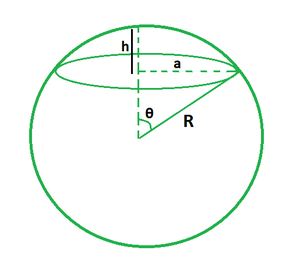Open in App
Not now

# Spherical Cap Volume Formula

• Last Updated : 27 Apr, 2022

A spherical cap is a part of a sphere that is obtained by cutting it with a plane. It is the section of a sphere that extends above the sphere’s plane and formed when a plane cuts off a part of a sphere. The base area, height, and sphere radius are all the values that are required to calculate the volume of a spherical cap.Formula

V = (1/3)π(3R – h)h2

where,

R is the radius of sphere,

h is the height of spherical cap,

π is a constant with a value of 22/7.

Using Pythagoras theorem, we can say that (R – h)2 + a2 = R2. So, the formula can also be written as,

V = (1/6)πh(3a2 + h2)

Here, a denotes the radius of spherical cap.

### Sample Problems

Problem 1. Find the volume of the spherical cap if the radius of the sphere is 7 m and the height of the cap is 10 m.

Solution:

We have, r = 7 and h = 10.

Using the formula we have,

V = (1/3)π(3R – h)h2

= (1/3) (22/7) (3(7) – 10) (102)

= (1/3) (22/7) (11) (100)

= 1152 cu. m

Problem 2. Find the volume of the spherical cap if the radius of the sphere is 5 m and the height of the cap is 5 m.

Solution:

We have, r = 5 and h = 5.

Using the formula we have,

V = (1/3)π(3R – h)h2

= (1/3) (22/7) (3(5) – 5) (52)

= (1/3) (22/7) (10) (25)

= 261.8 cu. m

Problem 3. Find the volume of the spherical cap if the radius of the sphere is 7.5 m and the height of the cap is 12 m.

Solution:

We have, r = 7.5 and h = 12.

Using the formula we have,

V = (1/3)π(3R – h)h2

= (1/3) (22/7) (3(7.5) – 5) (122)

= (1/3) (22/7) (17.5) (144)

= 1583.4 cu. m

Problem 4. Find the radius of the sphere if the height and volume of the cap are 15 m and 2120.6 cu. m respectively.

Solution:

We have, V = 2120.6 and h = 15.

Using the formula we have,

V = (1/3)π(3R – h)h2

=> 2120.6 = (1/3) (22/7) (3R – 15) (152)

=> 2120.6 = (1/3) (22/7) (3R – 15) (225)

=> 3R – 15 = 9

=> 3R = 24

=> R = 8 m

Problem 5. Find the radius of the spherical cap if the height and volume of the cap are 6.5 m and 1305.2 cu. m respectively.

Solution:

We have, V = 1305.2 and h = 6.5.

Using the formula we have,

V = (1/3)π(3R – h)h2

=> 1305.2 = (1/3) (22/7) (3R – 15) (6.5)2

=> 1305.2 = (1/3) (22/7) (3R – 6.5) (42.25)

=> 3R – 6.5 = 29.5

=> 3R = 36

=> R = 12 m

Now using the formula (R – h)2 + a2 = R2, we have

a2 = R2 – (R – h)2

a2 = 122 – (12 – 6.5)2

a2 = 144 – 30.25

a2 = 113.75

a = 10.67 m

Problem 6. Find the volume of a spherical cap if its radius is 7 m and height is 14 m.

Solution:

We have, a = 7 and h = 14.

Using the formula we have,

V = (1/6)πh(3a2 + h2)

= (1/6) (22/7) (14) (3 (7)2 + 142)

= (1/6) (22/7) (14) (343)

= 2514.3 cu. m

Problem 7. Find the volume of a spherical cap if its radius is 4.21 m and height is 9.54 m.

Solution:

We have, a = 4.21 and h = 9.54.

Using the formula we have,

V = (1/6)πh(3a2 + h2)

= (1/6) (22/7) (9.54) (3 (4.21)2 + 9.542)

= (1/6) (22/7) (14) (144.183)

= 720.2 cu. m

My Personal Notes arrow_drop_up
Related Articles